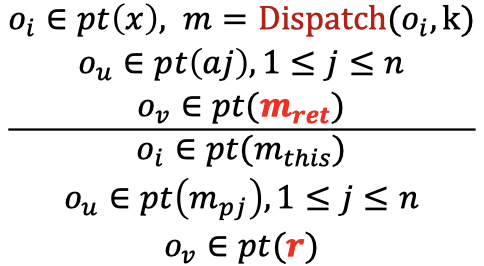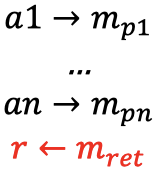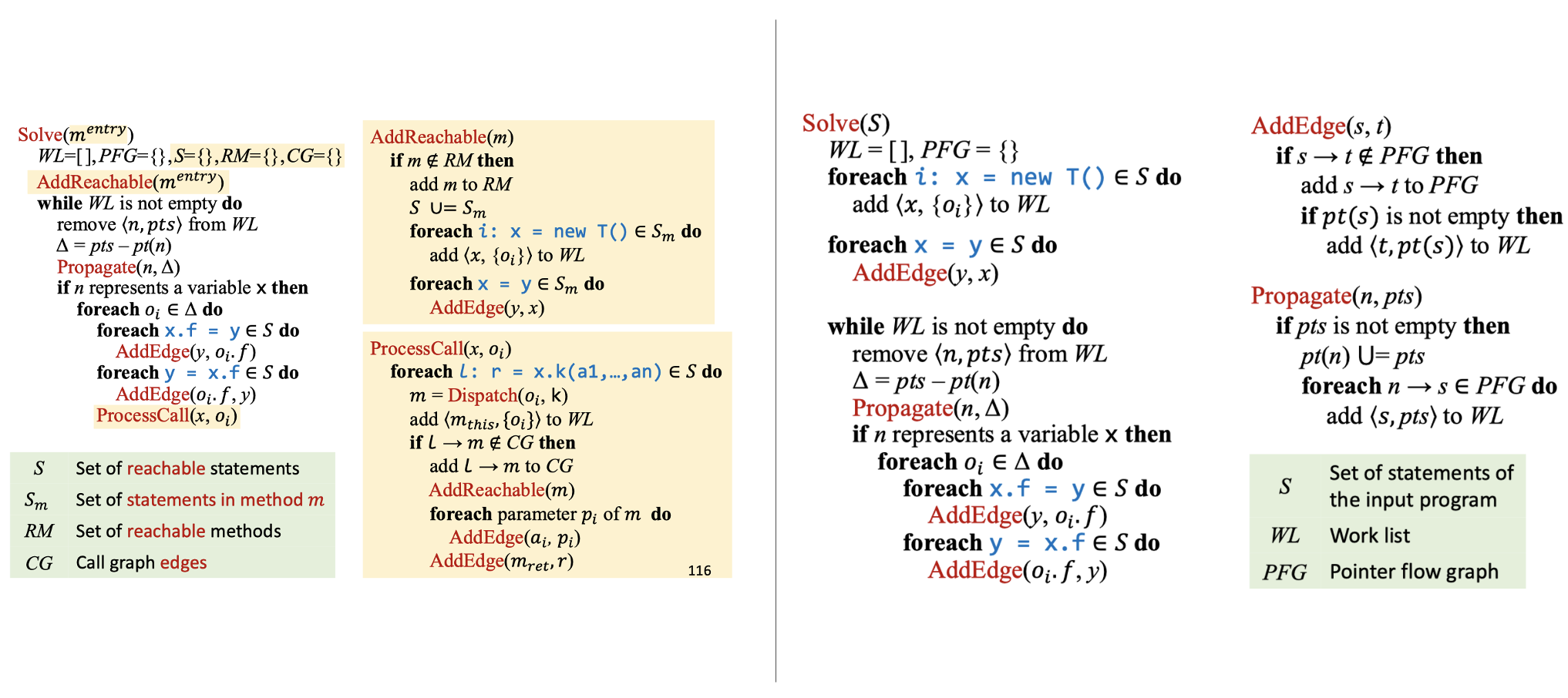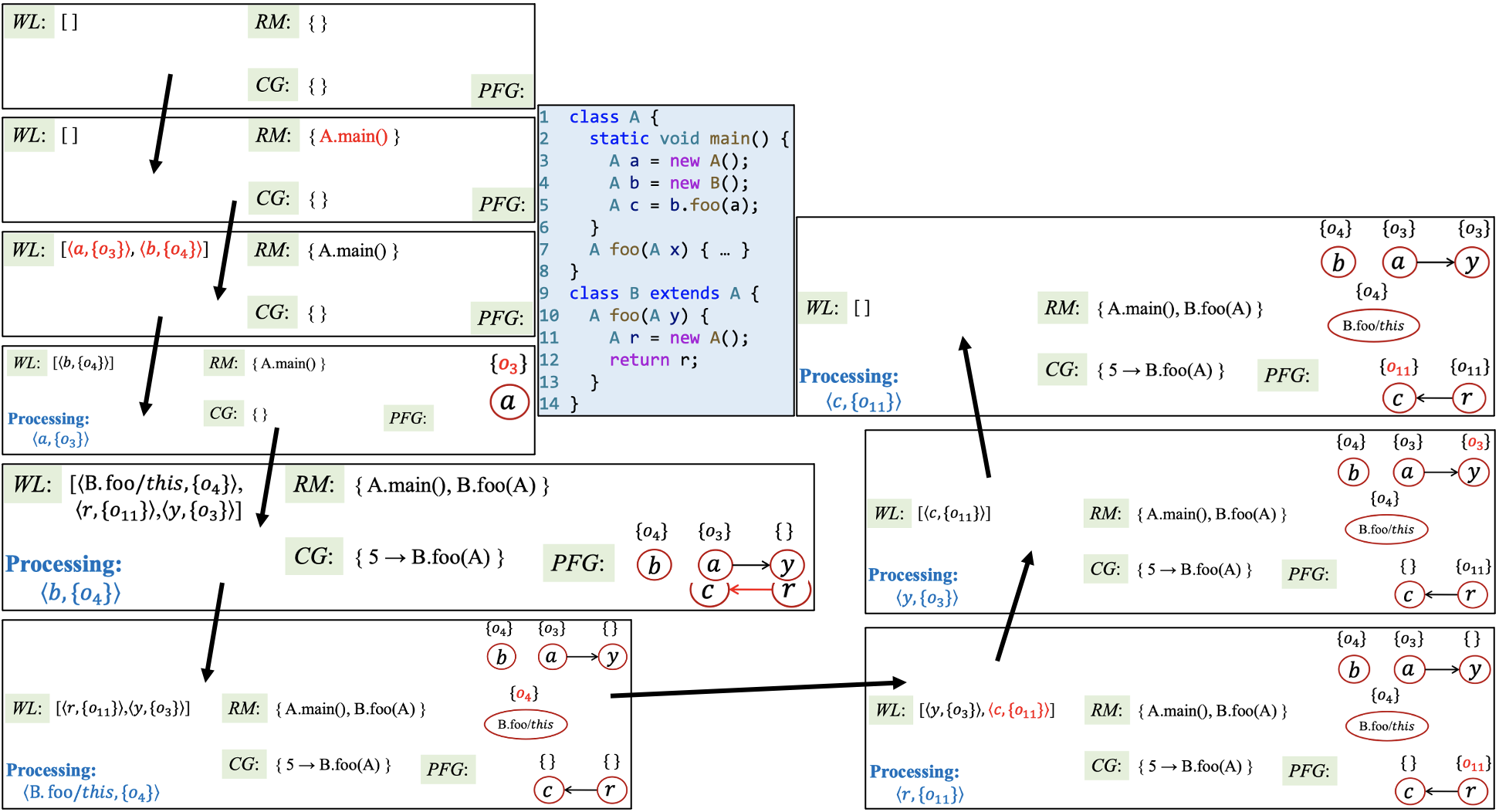## 方法调用的指针分析规则

New i: x = new T() $\frac{}{o_{i} \in pt(x)}$ N/A
Assign x = y $\frac{o_{i} \in pt(y)}{o_{i} \in pt(x)}$ $x \leftarrow y$
Store x.f = y $\frac{o_{i} \in pt(x),\ o_{j} \in pt(y)}{o_{j} \in pt(o_{i}.f)}$ $o_{i}.f \leftarrow y$
Load y = x.f $\frac{o_{i} \in pt(x),\ o_{j} \in pt(o_{i}.f)}{o_{j} \in pt(y)}$ $y \leftarrow o_{i}.f$
Call l: r = x.k(a1, ..., an)## 过程间指针分析